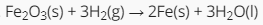# Stoichiometry and its Applications

This quiz will give you some more practice in solving the various kinds of stoichiometric calculations. Remember that you cannot solve the questions without a balanced chemical equation (none will be provided this time) and the appropriate mole ratio. Review your notes and use them to help you answer the following questions. You will also need access to a periodic table and a calculator. Select the best answer from the choices.

Start Quiz

Ammonia and copper(II) oxide react to form solid copper, water, and nitrogen gas. What are the coefficients for the balanced chemical equations?

2,3,2,3,1

1,3,3,3,1

1,0,3,3,1

2,3,3,3,2

If 40g of ammonia reacts with 80.0g copper(II) oxide, determine the limiting reactant.

Ammmonia

Hydrogen

Copper(II) oxide

Nitrogen

Determine the mass of nitrogen gas produced in the reaction.

23g

8.9g

9.44g

10.2g

When copper(II) oxide is heated with hydrogen gas, water and copper metal is produced. What mass of copper can be obtained if 32.0g of copper(II) oxide is used?

15.5g

25.5g

25g

35.5g

Nitrogen oxide reacts with oxygen gas to produce nitrogen dioxide. What are the coefficients that balance the equation?

2,1,2

1,1,2

1,1,1

2,2,1

How many moles of Fe can be made from 6 moles H2?4

3

5

7

About how many grams of H2O will be produced from 150 grams of Fe2O3?

500g

50g

200g

70g

What mass of CO2 will be produced from the reaction of 37.5 g C2H6?

320g

55g

345g

110g

Quiz/Test Summary
Title: Stoichiometry and its Applications
Questions: 8
Contributed by: